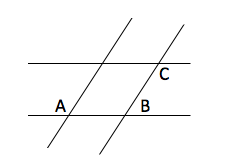# ACT Mathematics: Algebra and Exponent Quiz

This is an MCQ-based quiz on the topic of ACT Mathematics: Algebra and Exponent Quiz.

This set of MCQs helps you brush up on the important mathematics topic and prepare you to dive into skill practice and expand your knowledge to tackle the question and answer it carefully.

Start Quiz

Maria is planning the seating for the head table at a college gala. There are eight speakers that will be seated along one side of the table. Richard wants to sit beside Hang, and Maria knows that Thomas and Lily should not be seated together. In how many ways can Maria make up the seating plan?

7200

3975

10080

8100

If ∠A measures (40−10x)∘, which of the following is equivalent to the measure of the supplement of ∠A ?

10x + 50

100x

140 - 10x

10x + 140

Two pairs of parallel lines intersect:If A = 135o, what is 2*|B-C| = ?

180

140

150

170

Evaluate

(x^3)^2 = x^___

23

6

5

9

Evaluate:

–1 + 2 * –3

7

-7

5

3

Evaluate:

10 – 11 * (–1)^2

-21

1

21

-1

Evaluate:

10 – 11 * (–1)^2

-21

1

21

-1

Evaluate the expression:

(2 + 2)^2 – 1

1

7

4

15

The average of a list of 4 numbers is 90.0. A new list of 4 numbers has the same first 3 numbers as the original list, but the fourth number in the original list is 80, and the fourth number in the new list is 96. What is the average of this new list of numbers?

90.0

91.5

94.0

94.5

At the school carnival, Mike will play a game in which he will toss a penny, a nickel, and a dime at the same time. He will be awarded 3 points for each coin that lands with heads faceup. Let the random variable x represent the total number of points awarded on any toss of the coins. What is the expected value of x ?

1

3/2

9/2

6

Quiz/Test Summary
Title: ACT Mathematics: Algebra and Exponent Quiz
Questions: 10
Contributed by: